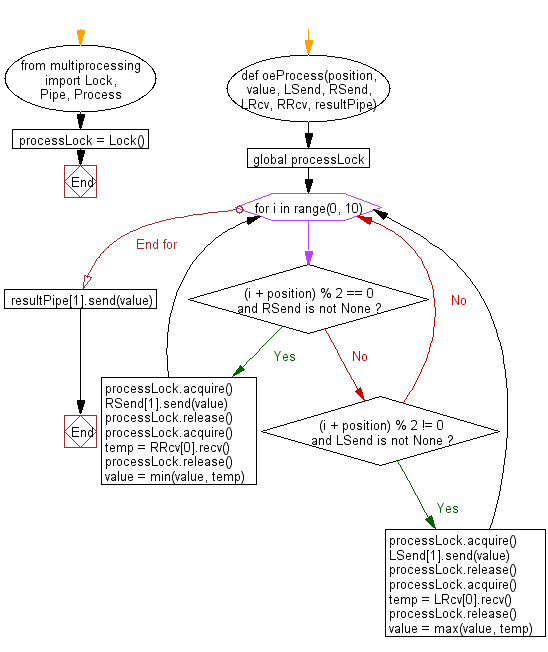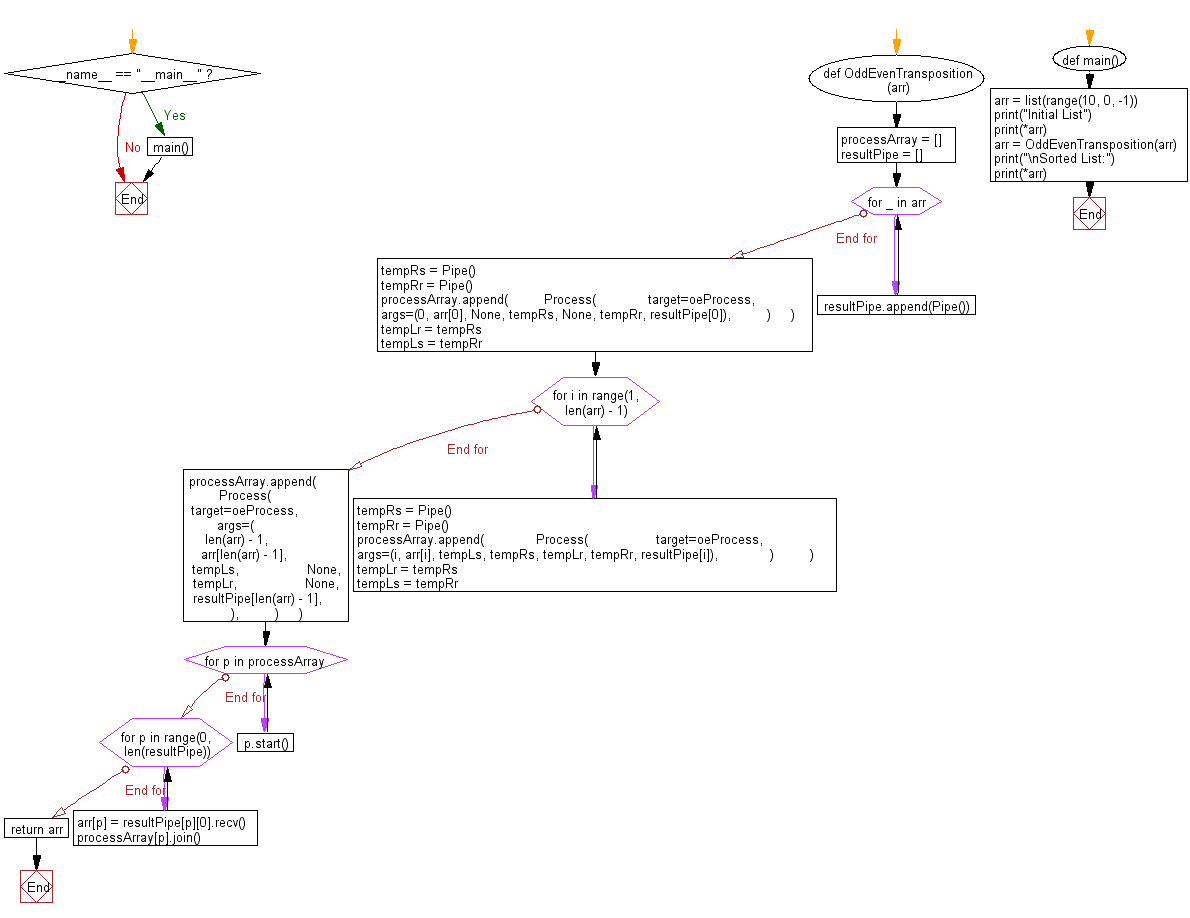﻿ Python: Sort unsorted numbers using Odd Even Transposition Parallel sort - w3resource# Python: Sort unsorted numbers using Odd Even Transposition Parallel sort

## Python Search and Sorting : Exercise-37 with Solution

Write a Python program to sort unsorted numbers using Odd Even Transposition Parallel sort.

Odd Even Transposition Parallel sort:
This is an implementation of odd-even transposition sort.
It works by performing a series of parallel swaps between odd and even pairs of variables in the list.
This implementation represents each variable in the list with a process and each process communicates with its neighbouring processes in the list to perform comparisons.
They are synchronized with locks and message passing but other forms of synchronization could be used

Sample Solution:

Python Code:

``````#Ref.https://bit.ly/3cce7iB
from multiprocessing import Lock, Pipe, Process

# lock used to ensure that two processes do not access a pipe at the same time
processLock = Lock()
def oeProcess(position, value, LSend, RSend, LRcv, RRcv, resultPipe):
global processLock

# we perform n swaps since after n swaps we know we are sorted
# we *could* stop early if we are sorted already, but it takes as long to
# find out we are sorted as it does to sort the list with this algorithm
for i in range(0, 10):

if (i + position) % 2 == 0 and RSend is not None:
processLock.acquire()
RSend.send(value)
processLock.release()

processLock.acquire()
temp = RRcv.recv()
processLock.release()

# take the lower value since you are on the left
value = min(value, temp)
elif (i + position) % 2 != 0 and LSend is not None:
processLock.acquire()
LSend.send(value)
processLock.release()

processLock.acquire()
temp = LRcv.recv()
processLock.release()

# take the higher value since you are on the right
value = max(value, temp)
# after all swaps are performed, send the values back to main
resultPipe.send(value)
"""
the function which creates the processes that perform the parallel swaps
arr = the list to be sorted
"""
def OddEvenTransposition(arr):
processArray = []
resultPipe = []
# initialize the list of pipes where the values will be retrieved
for _ in arr:
resultPipe.append(Pipe())
# creates the processes
# the first and last process only have one neighbor so they are made outside
# of the loop
tempRs = Pipe()
tempRr = Pipe()
processArray.append(
Process(
target=oeProcess,
args=(0, arr, None, tempRs, None, tempRr, resultPipe),
)
)
tempLr = tempRs
tempLs = tempRr

for i in range(1, len(arr) - 1):
tempRs = Pipe()
tempRr = Pipe()
processArray.append(
Process(
target=oeProcess,
args=(i, arr[i], tempLs, tempRs, tempLr, tempRr, resultPipe[i]),
)
)
tempLr = tempRs
tempLs = tempRr

processArray.append(
Process(
target=oeProcess,
args=(
len(arr) - 1,
arr[len(arr) - 1],
tempLs,
None,
tempLr,
None,
resultPipe[len(arr) - 1],
),
)
)
# start the processes
for p in processArray:
p.start()
# wait for the processes to end and write their values to the list
for p in range(0, len(resultPipe)):
arr[p] = resultPipe[p].recv()
processArray[p].join()
return arr
# creates a reverse sorted list and sorts it
def main():
arr = list(range(10, 0, -1))
print("Initial List")
print(*arr)
arr = OddEvenTransposition(arr)
print("\nSorted List:")
print(*arr)
if __name__ == "__main__":
main()
```
```

Sample Output:

```Initial List
10 9 8 7 6 5 4 3 2 1

Sorted List:
1 2 3 4 5 6 7 8 9 10
```

Flowchart:Python Code Editor:

What is the difficulty level of this exercise?

Test your Programming skills with w3resource's quiz.

﻿

## Python: Tips of the Day

Use Reversed() In for Loops:

```>>> tasks = ['laundry', 'picking up kids', 'gardening', 'cooking']